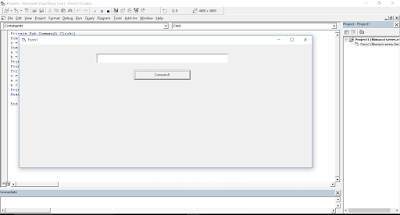# Visual Basic 6 Fibonacci Series

## Visual Basic 6 Fibonacci Series

Guys..
We are going to generate a Fibonacci sequence in Vb.Fibonacci series is the series in the first term starts from 0 and next term will be the addition of the previous two terms.

we will design the form in which we will take one text box for taking the input up-to which the series will be generated and one command button.we will write the code under the click event of the command button.Private Sub Command1_Click( )

Dim  n, a, b, c as Integer
n = val(Text1.Text)
a =0
b =1
Print a
Print b
For i = 1 To n - 2
c = a + b
a = b
b = c
Print c
Next i
End Sub

NOTE:

1.The program uses if next loop structure.
2.Dim is used to declared a variable.
3.Val function is used to change a string to numeric.